Journal topic
Solid Earth, 11, 1423–1440, 2020
https://doi.org/10.5194/se-11-1423-2020Special issue: Faults, fractures, and fluid flow in the shallow crust

Solid Earth, 11, 1423–1440, 2020
https://doi.org/10.5194/se-11-1423-2020Research article 28 Jul 2020

Research article | 28 Jul 2020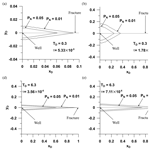# Bilinear pressure diffusion and termination of bilinear flow in a vertically fractured well injecting at constant pressure

Bilinear pressure diffusion and termination of bilinear flow in a vertically fractured well injecting at constant pressure
Patricio-Ignacio Pérez Donoso1, Adrián-Enrique Ortiz Rojas2, and Ernesto Meneses Rioseco3,a Patricio-Ignacio Pérez Donoso et al.
• 1Department of Mechanical Engineering, Universidad Técnica Federico Santa María, Valparaíso, 2340000, Chile
• 2Department of Chemical and Environmental Engineering, Universidad Técnica Federico Santa María, Valparaíso, 2340000, Chile
• 3Department of Geothermics and Information Systems, Leibniz Institute for Applied Geophysics, 30655 Hanover, Germany
• acurrently at: Department of Subsurface Use, Federal Institute for Geosciences and Natural Resources, 30655 Hanover, Germany

Abstract

This work studies intensively the flow in fractures with finite hydraulic conductivity intersected by a well injecting or producing at constant pressure, either during an injection or production well test or the operation of a production well. Previous investigations showed that for a certain time the reciprocal of flow rate is proportional to the fourth root of time, which is characteristic of the flow regime known as bilinear flow. Using a 2D numerical model, we demonstrated that during the bilinear flow regime the transient propagation of isobars along the fracture is proportional to the fourth root of time. Moreover, we present relations to calculate the termination time of bilinear flow under constant injection or production well pressure as well as an expression for the bilinear hydraulic diffusivity of fractures with finite hydraulic conductivity. To determine the termination of bilinear flow regime, two different methods were used: (a) numerically measuring the transient flow rate in the well and (b) analyzing the propagation of isobars along the fracture. Numerical results show that for low dimensionless fracture conductivities the transition from bilinear flow to another flow regime (e.g., pseudo-radial flow) occurs before the pressure front reaches the fracture tip, and for high dimensionless fracture conductivities it occurs when the pressure front arrives at the fracture tip. Hence, this work complements and advances previous research on the interpretation and evaluation of well test analysis under different reservoir conditions. Our results aim to improve the understanding of the hydraulic diffusion in fractured geologic media, and as a result they can be utilized for the interpretation of hydraulic tests, for example to estimate the fracture length.

Highlights.

• The reciprocal of flow rate is proportional to the fourth root of time.

• The migration of isobars in the fracture is proportional to the fourth root of time.

• For low dimensionless fracture conductivities, bilinear flow ends before the pressure front reaches the fracture tip.

• For high dimensionless fracture conductivities, bilinear flow ends when the pressure front reaches the fracture tip.

• Isobars accelerate when they approach the fracture tip.

1 Introduction

Understanding the different flow regimes in fractured reservoirs has always been key in the interpretation and evaluation of hydraulic well tests as well as in the production optimization of reservoirs. An in-depth description of the behavior of multiple flow regimes in fractures is extremely important to master the physics behind the modeling and simulation and, hence, to reliably interpret the results. Models considering a double porosity were first examined by Barenblatt et al. (1960). They introduced the basics of fluid dynamics in fissured rocks by deriving general equations of the seepage of liquid in porous media, taking into consideration its double-porosity condition. Cinco-Ley and Samaniego-V. (1981) differentiated clearly four flow regimes: fracture linear flow, bilinear flow (for the first time named in this way by them), formation linear flow, and pseudo-radial flow.

Usually, reservoir properties are obtained from well test or production data at a constant flow rate (pressure transient analysis). However, in most cases, reservoir production is performed at a constant pressure. This is illustrated, for instance, by the case where fluid is produced from the reservoir by means of a separator or constant-pressure pipeline (e.g., gas wells; Ehlig-Economides, 1979). Open wells flow at constant atmospheric pressure, e.g., artesian water wells. Geothermal fluid production may propel a back-pressure steam turbine, where steam leaves the turbine at the atmospheric pressure or at a higher constant pressure. Other operational conditions that require maintaining a constant pressure are encountered in gas wells, where a fixed pressure must be maintained for sales purposes, or in water injection wells, where the injection pressure is constant (Da Prat, 1990). In addition, reservoir production at constant pressure is conducted during rate decline periods of reservoir depletion (Da Prat, 1990; Ehlig-Economides, 1979). Although the interpretation of data collected in well tests and production at a constant flow rate (pressure transient analysis) has considerably improved, the rate transient analysis has not experienced such development (Houzé et al., 2018). Lately, a significant interest in the rate transient analysis has increased, which is attributed to the exploitation of unconventional hydrocarbon plays due to the extremely slow and long transient responses (Houzé et al., 2018). The production from unconventional plays has recently been made possible by creating fractures, which has strengthened the importance of having better tools and methods that allow us to obtain information on the fractures considering either the transient analysis of pressure or flow rate or the combination of both. It is exceedingly difficult to maintain a constant flow rate over long times, especially in low-permeability formations as in the case of unconventional plays (Kutasov and Eppelbaum, 2005). It is worth mentioning that constant-pressure tests have the advantage of minimizing changes in the wellbore storage coefficient (Earlougher Jr., 1977). The wellbore storage effects distort early-time pressure evolution; subsequently, the constant-pressure well tests allow the analysis of early-time data, and in this way information of the reservoir in the vicinity of the wellbore can be obtained (Nashawi and Malallah, 2007). Moreover, rate-transient tests are particularly suitable for the illustration of the long-term behavior of formations (Torcuk et al., 2013). Conceivably, one of the main reasons why constant-pressure tests are not a more common technique in reservoir engineering is that no analytical solutions are available for the pressure diffusivity equation when considering injection or production at constant pressure in fractured geologic media (Kutasov and Eppelbaum, 2005).

Arps (1945) presented an empirical production correlation for the rate history of a well during the boundary-dominated flow regime. Later, Locke and Sawyer (1975) generated type curves for a vertically fractured reservoir producing at constant pressure with the objective of characterizing the behavior of flow rate. In this context, Agarwal et al. (1979) presented type curves to analyze the early-time cases. In addition, they determined the dimensionless fracture conductivity TD by means of graphing the logarithm of the reciprocal of flow rate vs. the logarithm of time and utilizing type-curve matching techniques (please also see the table in the Appendix for a list of symbols used in this paper). Fetkovich (1980) introduced the rate decline analysis in the radial-flow system, similar to pressure transient analysis; however, it was only applicable to circular homogeneous reservoirs.

Guppy et al. (1981a) studied the effect of non-Darcy flow within a fracture. They concluded that the dimensionless fracture conductivity TD can be expressed as an apparent conductivity that is not constant over time. Subsequently, a major contribution was made by Guppy et al. (1981b), which consisted of presenting semi-analytical solutions for bilinear flow; both works considered constant-pressure production. They demonstrated that the reciprocal of dimensionless flow rate is proportional to the fourth root of dimensionless time when producing at constant wellbore pressure. Guppy et al. (1988) contributed further to the previous works investigating thoroughly the cases with turbulent flow in the fracture, and for the first time they examined a technique that concerns both buildup and drawdown data when the well is producing at constant pressure. Subsequently, a direct method to estimate the turbulent term considering high-velocity flow in variable rate tests was documented by Samaniego-V. and Cinco-Ley (1991). In addition, Berumen et al. (1997) developed a transient pressure analysis under both constant wellhead and bottom-hole pressure conditions considering high-velocity flow. Wattenbarger et al. (1998) presented decline curve analysis methods for tight gas wells producing at constant pressure with long-term linear behavior (fracture flow). Pratikno et al. (2003) prepared rate–time decline curves for fractured wells producing at constant pressure, including fracture lineal and bilinear flow. Follow-up investigations conducted by Nashawi (2006) presented semi-analytical solutions when considering non-Darcy flow in a fracture and a method with which it is possible to quantify the turbulence in a fracture. Nashawi and Malallah (2007) developed a direct method to determine the fracture and reservoir parameters without having to use type-curve matching techniques. In this context, Heidari Sureshjani and Clarkson (2015) concluded that plotting techniques overestimate the fracture half-length, leading them to the formulation of an analytical methodology with which the fracture half-length is estimated more precisely.

Recently, Silva-López et al. (2018) introduced a new method to obtain Laplace-transform solutions, and as a result, they predicted new regions of flow behavior. This latter method is documented for injection at either constant flow rate or pressure. In addition, the theory of well testing has been improved by investigating the effects of non-uniform properties of hydraulic fractures (He et al., 2018). Moreover, Wang et al. (2018) presented an enhanced model to simulate the productivity of volume fractured wells and Dejam et al. (2018) documented a new semi-analytical solution applicable in dual-porosity formulations.

When it comes to studying the termination of bilinear flow regime and the spatiotemporal propagations of isobars, there is not much evidence of investigations considering injection or production at constant pressure. To the best of our knowledge, it has only been investigated when injecting at a constant flow rate in the well (Cinco-Ley and Samaniego-V., 1981; Weir, 1999). In this regard, new criteria to determine the end of bilinear flow, which are also used in this investigation, were introduced by Ortiz R. et al. (2013). From the industry point of view, accurately estimating the termination time of the bilinear flow is relevant since it can be used to assess a minimum value of fracture length when the dimensionless fracture conductivity TD≥3 (Cinco-Ley and Samaniego-V., 1981). To underpin the latter, Ortiz R. et al. (2013) demonstrated that for TD approximately higher than 10 the fracture half-length can be estimated as ${x}_{\mathrm{F}}=C{\left({D}_{\mathrm{b}}{t}_{\mathrm{ebl}}\right)}^{\mathrm{1}/\mathrm{4}}$, where C is a constant, Db is the bilinear hydraulic diffusivity, and tebl the termination time of the bilinear flow. Moreover, for lower values of TD the termination time of the bilinear flow can be used to restrict the minimum fracture length. This information is important to characterize and model a fractured reservoir. Having reliable data on fracture dimensions is critically important for production optimization strategies.

This work addresses the challenging task of gaining a quantitative understanding of bilinear flow from rate transient analysis for wells producing at constant pressure, requiring a multidisciplinary approach. Expanding the understanding of bilinear flow regime in fractured reservoirs leads to a more precise analysis of well tests and production or injection data. This, in turn, makes it possible to characterize a reservoir more accurately and consequently have more reliable assessments of its behavior, leading to better concepts of production optimization during operation. Some of the methodologies used in this work are inspired by the study conducted by Ortiz R. et al. (2013) for wells operating at a constant flow rate (pressure transient analysis).

Taking into account injection at constant pressure, this investigation presents for the first time: (a) the propagation of isobars PN along the fracture and the formation during bilinear flow regime, as well as the computation of the bilinear hydraulic diffusivity of fracture, and (b) the study of termination of bilinear flow regime utilizing criteria previously presented and a criterion firstly documented here.

2 Background

## 2.1 Governing equations and parameters

This study is carried out considering that single-phase fluid in both matrix and fracture obeys Darcy's law in a two-dimensional confined and saturated aquifer. In a general formulation of a dual-porosity dual-permeability model, the equation utilized to describe the hydraulics of a single-phase compressible Newtonian fluid in a reservoir matrix is given by

$\begin{array}{}\text{(1)}& {s}_{\mathrm{m}}\frac{\partial p}{\partial t}=\frac{{k}_{\mathrm{m}}}{{\mathit{\eta }}_{\mathrm{f}}}\left(\frac{{\partial }^{\mathrm{2}}p}{\partial {x}^{\mathrm{2}}}+\frac{{\partial }^{\mathrm{2}}p}{\partial {y}^{\mathrm{2}}}\right),\end{array}$

where sm (Pa−1) represents the specific storage capacity of matrix, km (m2) the matrix permeability, ηf (Pa s) the dynamic fluid viscosity, and p (Pa) the fluid pressure. It is worth noting that the storage coefficient depends on the porosity of rock and compressibility of fluid and rock. For the fracture, the equation is given by

$\begin{array}{}\text{(2)}& {s}_{\mathrm{F}}{b}_{\mathrm{F}}\frac{\partial p}{\partial t}=\frac{{T}_{\mathrm{F}}}{{\mathit{\eta }}_{\mathrm{f}}}\frac{{\partial }^{\mathrm{2}}p}{\partial {x}^{\mathrm{2}}}+\frac{{q}_{\mathrm{F}}\left(x,t\right)}{h},\end{array}$

where sF (Pa−1) represents the specific storage capacity of fracture, bF (m) the aperture of fracture, TF (m3) the fracture conductivity, h (m) the fracture height, and qF(x,t) the fluid flow between matrix and fracture (see Cinco L. et al., 1978; Guppy et al., 1981b). In this study, sF is neglected because we assume that the fracture is non-deformable and the amount of fluid in the fracture is small enough to consider its compressibility as negligible. In addition, the porosity of the fracture is negligible in comparison to the porosity of the matrix. Note, however, that the pressure in the fracture is dictated by an inhomogeneous diffusivity equation, which contains a time-dependent source term qF(x,t), but it does not involve an intrinsic transient term. Thus, Eq. (2) reads

$\begin{array}{}\text{(3)}& \frac{{T}_{\mathrm{F}}}{{\mathit{\eta }}_{\mathrm{f}}}\frac{{\partial }^{\mathrm{2}}p}{\partial {x}^{\mathrm{2}}}+\frac{{q}_{\mathrm{F}}\left(x,t\right)}{h}=\mathrm{0}.\end{array}$

The pressure diffusivity equations for matrix and fracture are coupled by the term qF(x,t), which is defined as

$\begin{array}{}\text{(4)}& \frac{{q}_{\mathrm{F}}\left(x,t\right)}{h}=\mathrm{2}\frac{{k}_{\mathrm{m}}}{{\mathit{\eta }}_{\mathrm{f}}}{\frac{\mathrm{d}p}{\mathrm{d}y}|}_{y=\mathrm{0}},\end{array}$

where the factor 2 relates to the contact between matrix and fracture via its two surfaces.

## 2.2 Dimensionless parameters

This study is conducted using dimensional properties, but the analysis of results is performed utilizing the conventional dimensionless definitions. The dimensionless flow rate is given by

$\begin{array}{}\text{(5)}& \frac{\mathrm{1}}{{q}_{\mathrm{wD}}}=\frac{{k}_{\mathrm{m}}h\left({p}_{\mathrm{w}}-{p}_{\mathrm{i}}\right)}{{q}_{\mathrm{w}}{\mathit{\eta }}_{\mathrm{f}}},\end{array}$

where qw (m3 s−1) represents the flow rate in the well, qwD the dimensionless flow rate in the well, h (m) the fracture height, pi (Pa) the initial pressure of the formation and fracture, and pw (Pa) the constant injection pressure.

The dimensionless fracture conductivity is defined as

$\begin{array}{}\text{(6)}& {T}_{\mathrm{D}}=\frac{{T}_{\mathrm{F}}}{{k}_{\mathrm{m}}{x}_{\mathrm{F}}},\end{array}$

where TF=kFbF (m3) denotes the fracture conductivity, xF (m) the fracture half-length, and kF (m2) the fracture permeability. Note that TD is the same as ${\left({k}_{\mathrm{f}}{b}_{\mathrm{f}}\right)}_{\mathrm{D}}$ used in Cinco-Ley and Samaniego-V. (1981) or FCD used in Gidley et al. (1990).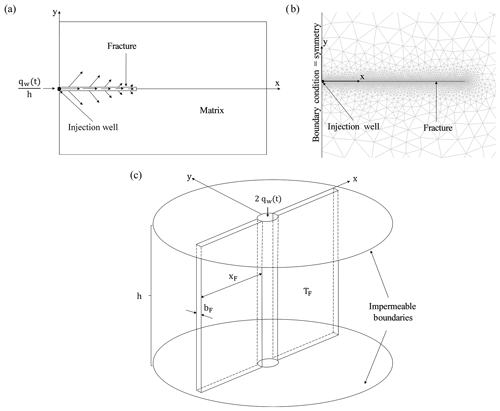Figure 1(a) Two-dimensional representation of model structure; (b) mesh utilized for simulation; and (c) 3D representation of model structure.

Instead of using the conventional definition of dimensionless time ${t}_{\mathrm{D}}=t{D}_{\mathrm{m}}/{x}_{\mathrm{F}}^{\mathrm{2}}$, we prefer to use a modified definition presented by Ortiz R. et al. (2013):

$\begin{array}{}\text{(7)}& \mathit{\tau }=\frac{{t}_{\mathrm{D}}}{{T}_{\mathrm{D}}^{\mathrm{2}}}=\frac{{D}_{\mathrm{m}}{k}_{\mathrm{m}}^{\mathrm{2}}}{{T}_{\mathrm{F}}^{\mathrm{2}}}t,\end{array}$

where ${D}_{\mathrm{m}}={k}_{\mathrm{m}}/\left({\mathit{\eta }}_{\mathrm{f}}{s}_{\mathrm{m}}\right)$ (m2 s−1) represents the hydraulic diffusivity of matrix and τ the dimensionless time. Finally, the dimensionless x coordinate, which corresponds to the fracture axis (see Fig. 1), is defined as

$\begin{array}{}\text{(8)}& {x}_{\mathrm{D}}=\frac{x}{{x}_{\mathrm{F}}};\end{array}$

and the dimensionless y coordinate, which represents the axis perpendicular to the fracture (see Fig. 1), is defined as

$\begin{array}{}\text{(9)}& {y}_{\mathrm{D}}=\frac{y}{{x}_{\mathrm{F}}}.\end{array}$

## 2.3 Previous solutions for bilinear flow at a constant wellbore pressure

As mentioned earlier, a bilinear flow regime was firstly documented by Cinco-Ley and Samaniego-V. (1981). According to their proposed definition, it consists of an incompressible linear flow within the fracture and a slightly compressible linear flow in the formation. Moreover, a semi-analytical solution for a vertically fractured well producing at constant pressure during bilinear flow regime was presented by Guppy et al. (1981b). They demonstrated that the reciprocal of flow rate is proportional to the fourth root of time and the governing equation is given in dimensionless form by

$\begin{array}{}\text{(10)}& \frac{\mathrm{1}}{{q}_{\mathrm{wD}}}\left(\mathit{\tau }\right)=\frac{\mathit{\pi }\mathrm{\Gamma }\left(\mathrm{3}/\mathrm{4}\right)}{\sqrt{\mathrm{2}{T}_{\mathrm{D}}}}{t}_{\mathrm{D}}^{\mathrm{1}/\mathrm{4}}\cong \mathrm{2.722}{\mathit{\tau }}^{\mathrm{1}/\mathrm{4}},\end{array}$

where Γ(3∕4) represents the gamma function evaluated in 3∕4. Silva-López et al. (2018) presented an analytical solution for an infinite fracture considering the case of variable flow rate for a long time in dimensionless form:

$\begin{array}{}\text{(11)}& \frac{\mathrm{1}}{{q}_{\mathrm{wD}}}\left({t}_{\mathrm{D}}\right)=\frac{\mathrm{1}}{f\left({t}_{\mathrm{D}}\right)}\frac{{\mathit{\pi }}^{\mathrm{1}/\mathrm{4}}\sqrt{{T}_{\mathrm{D}}}}{\mathrm{2}\mathit{\delta }}{t}_{\mathrm{D}}^{\mathrm{1}/\mathrm{4}}.\end{array}$

Note that Eq. (11) is written in the notation used in this paper. f(tD) represents a function that describes the transient behavior of pressure in the well and δ denotes a constant.

## 2.4 Description of the model setup

We ran the numerical simulations in the Subsurface Flow Module of COMSOL Multiphysics® software program. The space- and time-dependent balance equations, described in Sect. 2.1, together with their initial and boundary conditions are numerically solved in the entire modeling domain employing the finite-element method (FEM) in a weak formulation. The discretization of the partial differential equations (PDEs) results in a large system of sparse linear algebraic equations, which are solved using the linear system solver MUMPS (MUltifrontal Massively Parallel Sparse direct Solver), implemented in the finite-element simulation software COMSOL Multiphysics®. Utilizing the Galerkin approach, Lagrange quadratic shape functions have been selected to solve the discretized diffusivity equations for the pressure process variable. For the time discretization, a backward differentiation formula (BDF, implicit method) of variable order has been chosen.

The two-dimensional model setup in this work is composed of a vertical fracture embedded in a confined horizontal reservoir. The matrix and fracture are porous geologic media considered saturated, continuous, isotropic, and homogeneous. The gravity effects are neglected. Fluid flow enters or abandons the matrix–fracture system only through the well. This investigation is symmetric, i.e., the flow rate calculated in the well qw corresponds to the half of total flow rate for the case of studying the complete fracture length (see Fig. 1). The pressure in the well pw is set to 1 MPa during the entire simulation and the initial conditions for pressure in the matrix and the fracture pi is set to 100 kPa. We use these pressure conditions in order to ensure an injection of fluid from the well to the matrix–fracture system. The order of magnitude of (pwpi) is similar to that utilized by Nashawi and Malallah (2007). No-flow boundary conditions are assigned to the boundaries of the reservoir since it is considered as confined. In order to ensure that the boundary conditions do not affect the modeling outcome, the system size was consecutively enlarged to double, triple, and quadruple, and the results were compared to each other and, in fact, they were identical. Additional studies have been conducted to further examine the independency of simulation results from the boundary conditions set for the simulation time considered. The pressure has been monitored at the boundary of the model for the case of imposing a no-flow boundary condition (closed reservoir). No pressure variation has been detected at the boundaries of the model, which corroborates the previous observation that the simulation results have not been affected by the boundary condition set. Further, the boundary condition has been changed to constant pressure (open reservoir). Also, for this latter case, no changes were recognized in the simulation results. That way, boundary condition independency of the solution has been guaranteed in the computational subdomain of the most interest. During the entire simulation the following parameters remained constant: km=1µD, ${k}_{\mathrm{F}}=\mathrm{1.5}×{\mathrm{10}}^{-\mathrm{13}}$ m2, ${s}_{\mathrm{m}}=\mathrm{1}×{\mathrm{10}}^{-\mathrm{11}}$ Pa−1, ${b}_{\mathrm{F}}=\mathrm{1}×{\mathrm{10}}^{-\mathrm{3}}$ m, and ${\mathit{\eta }}_{\mathrm{f}}=\mathrm{2.5}×{\mathrm{10}}^{-\mathrm{4}}$ Pa s. Similarly as in Ortiz R. et al. (2013), the fracture half-length takes different values from 1.5 m up to 1500 m, with the objective of varying the dimensionless fracture conductivity TD from 0.1 up to 100 (see Eq. 6). The time steps used in these numerical simulations were 0.01 s from the start until the first 40 s, 20 s from 40 until 600 s, 60 s from 600 until 12 000 s, 300 s from 12 000 until 72 000 s, 1000 s from 72 000 until 5×105 s, and 5×105 s from 5×105 until 2×108 s (or until 6×108 s employed for the master curve; Fig. 2).

The mesh, composed of triangular elements, is relatively fine in the vicinity of the fracture and the well, and it becomes gradually coarser when moving away from the fracture, since there is an extremely large hydraulic gradient near the fracture and the well (see Fig. 1b). The minimum element size is 0.0045 m near the well, the maximum element size is 80 m close to the boundaries of the reservoir, and the maximum element growth rate is 1.3 m. The number of elements varies according to the different size and mesh structure used to describe the respective model scenario. The minimum and maximum number of elements is 12 929 and 1 358 697, respectively. We performed mesh convergence studies refining the mesh, particularly, in the computational subdomain that contains steep hydraulic gradients, until the solution became mesh-independent.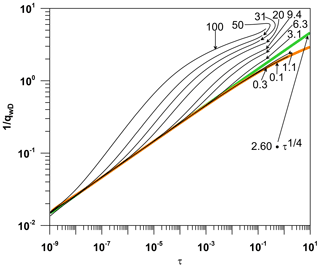Figure 2Model results displayed as 1∕qwD vs. τ on a log–log scale. Bilinear fit curve (green line), master curve (orange line), and type curves (black lines).

3 Results

Numerical simulations show that during a time interval, the reciprocal of dimensionless flow rate in the well 1∕qwD is proportional to the fourth root of dimensionless time τ1∕4 (Fig. 2). This proportionality is in accordance with the behavior documented by Guppy et al. (1981b) and Silva-López et al. (2018). In particular, we can describe the variation in dimensionless flow rate in the well during the bilinear flow regime as

$\begin{array}{}\text{(12)}& \frac{\mathrm{1}}{{q}_{\mathrm{wD}}}\left(\mathit{\tau }\right)=A\cdot {\mathit{\tau }}^{\mathrm{1}/\mathrm{4}},\end{array}$

where the constant A is equal to 2.60. From now on, we will refer to this equation as the bilinear fit curve (green line in Fig. 2). Note that the coefficient A obtained by Guppy et al. (1981b) employing a semi-analytical solution is approximately A=2.722 (see Eq. 10). This slight difference between our and their result for A might be due to the temporal and spatial discretization utilized by them. The reciprocal of dimensionless flow rate exhibits a behavior proportional to the fourth root of time (Eq. 12), which is characteristic of bilinear flow regime; hence we can corroborate the occurrence of it.

We define the master curve as the one that describes the behavior of an infinitely long fracture (orange line in Fig. 2). The curves describing the behavior of the reciprocal of dimensionless flow rate over time for different dimensionless fracture conductivities, from TD=0.1 up to TD=100, are addressed as type curves (black lines in Fig. 2).

Taking into account all the aspects previously described, when type curves start departing from the bilinear fit curve (Fig. 2), this indicates that the transition from bilinear flow regime to formation linear flow regime (cases with high TD) or to pseudo-radial flow regime (cases with low TD) begins (Ortiz R. et al., 2013).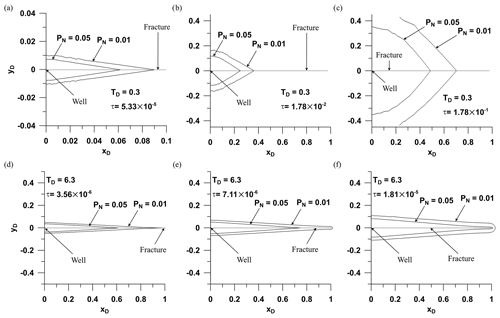Figure 3Spatial evolution of isobars PN=0.01 and PN=0.05 over time through the modeling domain, for the dimensionless fracture conductivities TD=0.3 (a, b, c) and TD=6.3 (d, e, f). Note that for the case of TD=0.3, the scale of the graph (a) is different from that used for the graphs (b) and (c). Read text in Sect. 3.1 for a more detailed description of the graphs.

## 3.1 Propagation of isobars along the fracture and the formation

In order to characterize the different isobars, the following definition is used (Ortiz R. et al., 2013):

$\begin{array}{}\text{(13)}& {P}_{\mathrm{N}}=\frac{p\left(x,y,t\right)-{p}_{\mathrm{i}}}{{p}_{\mathrm{w}}-{p}_{\mathrm{i}}},\end{array}$

where $p\left(x,y,t\right)$ denotes the pressure at the position (x,y) in the fracture or the matrix at time t. The values of PN utilized in this study are 0.01 and 0.05, which are equivalent to the isobars of 109 and 145 kPa, respectively. The isobars behave differently depending on the value of TD. For cases with low TD, it is noticeable that after the termination of bilinear flow, the isobars reveal a tendency of progressing toward an elliptical or pseudo-radial flow while still propagating along the fracture (see, for example, TD= 0.3 in Fig. 3a, b, c). The lower the value of TD, the more pronounced this tendency becomes. On the other hand, for high TD the behavior of the isobars is similar to the formation linear flow beyond the fracture (see TD=6.3 in Fig. 3d, e, f). Although the behavior of isobars after the termination of bilinear flow is also highly interesting, this aspect is not addressed in further detail in this work. It remains to be studied in a follow-up investigation.

The results of this investigation show that initially the migration of isobars PN along the fracture (see Fig. 1) is proportional to the fourth root of time:

$\begin{array}{}\text{(14)}& {x}_{\mathrm{iD}}={\mathit{\alpha }}_{\mathrm{b}}{T}_{\mathrm{D}}{\mathit{\tau }}^{\mathrm{1}/\mathrm{4}},\end{array}$

where xiD represents the dimensionless distance of normalized isobars PN from the well along the xD axis and αb is a constant that depends on the studied isobar PN (see Fig. 4).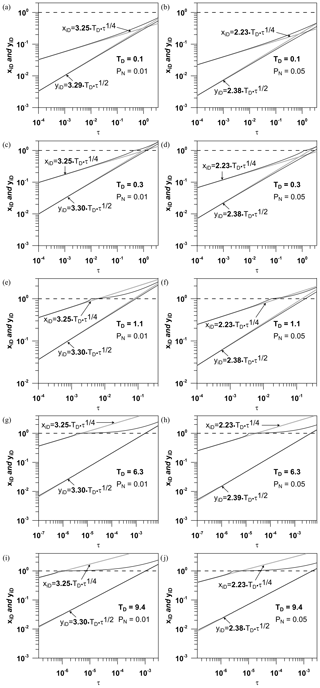Figure 4Model results display xiD and yiD vs. τ on a log–log scale. Propagation of isobars PN=0.01 (a, c, e, g, i) and PN=0.05 (b, d, f, h, j) along the fracture and the formation considering the following dimensionless fracture conductivities: TD=0.1 (a, b), 0.3 (c, d), 1.1 (e, f), 6.3 (g, h), and 9.4 (i, j). The dashed lines represent the arrival at the fracture tip of the specific isobars indicated in the graphs.

In addition, the migration of isobars PN in the matrix (perpendicular to the fracture and at xD=0; see Fig. 1) for short times may be described by

$\begin{array}{}\text{(15)}& {y}_{\mathrm{iD}}={\mathit{\alpha }}_{\mathrm{m}}{T}_{\mathrm{D}}{\mathit{\tau }}^{\mathrm{1}/\mathrm{2}},\end{array}$

where yiD denotes the dimensionless distance of normalized isobars PN from the well along the yD axis and αm is a constant for pressure diffusion in the matrix, which depends on the isobar under investigation.

When expressing Eqs. (14) and (15) in dimensional form, for the x axis, Eq. (14) is given by

$\begin{array}{}\text{(16)}& {x}_{\mathrm{i}}\left(t\right)={\mathit{\alpha }}_{\mathrm{b}}{\left({D}_{\mathrm{b}}t\right)}^{\mathrm{1}/\mathrm{4}};\end{array}$

and for the y axis, Eq. (15) is given by

$\begin{array}{}\text{(17)}& {y}_{\mathrm{i}}\left(t\right)={\mathit{\alpha }}_{\mathrm{m}}{\left({D}_{\mathrm{m}}t\right)}^{\mathrm{1}/\mathrm{2}}.\end{array}$

In Eq. (17), ${D}_{\mathrm{m}}={k}_{\mathrm{m}}/\left({\mathit{\eta }}_{\mathrm{f}}{s}_{\mathrm{m}}\right)$ (m2 s−1) is known as hydraulic diffusivity of matrix and is analogous to the definition of thermal diffusivity. Additionally, in Eq. (16) ${D}_{\mathrm{b}}={T}_{\mathrm{F}}^{\mathrm{2}}/{k}_{\mathrm{m}}{\mathit{\eta }}_{\mathrm{f}}{s}_{\mathrm{m}}$ (m4 s−1) is referred to as effective hydraulic diffusivity of fracture in a bilinear flow regime (Ortiz R. et al., 2013).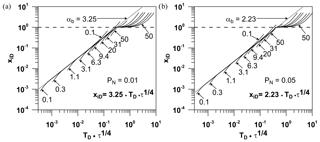Figure 5Model results in terms of xiD vs. ${T}_{\mathrm{D}}\cdot {\mathit{\tau }}^{\mathrm{1}/\mathrm{4}}$ on a log–log scale. Propagation of isobars PN along the fracture considering the following dimensionless fracture conductivities: TD=0.1, 0.3, 1.1, 3.1, 6.3, 9.4, 20, 31, and 50. (a) Model scenarios with PN=0.01 and αb=3.25; (b) model scenarios with PN=0.05 and αb=2.23. The dashed lines represent the arrival at the fracture tip of the specific isobars indicated in the graphs.

The numerical results are specified as migration type curves (see black lines in Figs. 4, 5, and 6), and the fit equations for the propagation of isobars are referred to as migration fit curves (see grey lines in Figs. 4, 5, and 6). It can be noted qualitatively throughout the cases under study that for low dimensionless fracture conductivities, i.e., TD=0.1 and TD=0.3, the migration type curves, which describe the migration of isobars PN along both the xD and the yD axis, start departing from migration fit curves before the studied isobars reach the fracture tip (Figs. 4a, b, c, d, and 5). In contrast, for the cases considering high dimensionless fracture conductivities, i.e., TD=1.1, TD=6.3, and TD=9.4, there is no qualitative evidence of migration type curves departing from migration fit curves before the studied isobars PN arrive at the fracture tip (Figs. 4e, f, g, h, i, j and 5). The latter results, however, show some exceptions for a slight acceleration exhibited by the isobars PN at times shortly before they reach the fracture tip. It is important to mention that this relatively small acceleration also occurs for cases with low dimensionless fracture conductivities (see Fig. 4c, d). The same behavior was observed by Ortiz R. et al. (2013) for the injection at a constant flow rate. The classic definition for acceleration was considered, which is the rate of change of velocity with respect to time.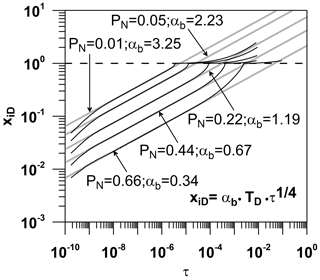Figure 6Modeling results in terms of xiD vs. τ on a log–log scale. Propagation of isobars PN=0.01, 0.05, 0.22, 0.44, and 0.66 with TD=6.3. The dashed line represents the arrival at the fracture tip of the specific isobars indicated in the graph.

On the one hand, when discussing the early time qualitatively, we notice that the higher the value of the isobar PN, the sooner it starts behaving proportional to the fourth root of time (Fig. 6). For example, at the same time ($\mathit{\tau }=\mathrm{5}\cdot {\mathrm{10}}^{-\mathrm{10}}$) the isobar PN=0.66 (the greater isobar under investigation) starts to migrate along the fracture proportional to the fourth root of time, whereas the isobar PN=0.01 (the smaller isobar under study) has not yet started to propagate proportionally to the fourth root of time. Moreover, the greater the isobar PN, the shorter its distance from the well xiD in comparison to other smaller isobars when considering the same time τ, which is logical since the isobars migrate one after the other. On the other hand, when discussing the long time qualitatively, we notice that the smaller isobar PN=0.01 departs from the migration fit curve when it reaches the fracture tip. In contrast, the greater isobar PN=0.66 departs from the migration fit curve before its arrival at the fracture tip (Fig. 6). Additionally, it can be seen that the higher the value of isobar PN, the farther from the fracture tip or, closer to the well, it starts departing from the migration fit curve. Thus, taking into consideration the migration of isobars, it is reasonable to conclude that for high dimensionless fracture conductivities TD, the bilinear flow regime ends when the pressure front reaches the fracture tip.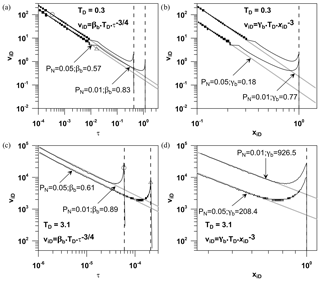Figure 7Model results showing viD vs. τ and viD vs. xiD on a log–log scale. Velocity of isobars PN=0.01 and 0.05 considering TD=0.3 (a, b) and 3.1 (c, d). The dashed lines represent the arrival of the specific isobars at the fracture tip. (a) The circle and triangle symbols represent the transition time τt for PN=0.01 and 0.05, respectively (see Eq. 20). (c) The circle and triangle symbols represent the arrival time τa for PN=0.01 and 0.05, respectively (see Fig. 8).

Previously, we referred to the observation concerning the acceleration that isobars experience at times shortly before they arrive at the fracture tip (see Figs. 4, 6), which was also documented in Ortiz R. et al. (2013) for the case of fluid injection at a constant flow rate. To prove that it is truly an acceleration, the velocity of isobars is determined by calculating ΔxiD∕Δτ, and it is graphed versus time τ as well as versus the distance of isobars from the well xiD (see Fig. 7). The existence of this acceleration in xiD=1 (fracture tip; see Fig. 7) can be clearly seen. The velocity of isobars viD during their migration along the fracture decreases almost for the complete intervals of time considered (Fig. 7a, c), except for its evident increase at times shortly before the isobars reach the fracture tip. The velocity of isobars can be described within the intervals of time used as

$\begin{array}{}\text{(18)}& {v}_{\mathrm{iD}}\left(\mathit{\tau }\right)={\mathit{\beta }}_{\mathrm{b}}{T}_{\mathrm{D}}{\mathit{\tau }}^{-\mathrm{3}/\mathrm{4}},\end{array}$

where βb is a constant that depends on the isobar under study. The velocity of isobars in terms of their distances from a well and within the ranges of distance used can be described as

$\begin{array}{}\text{(19)}& {v}_{\mathrm{iD}}\left({x}_{\mathrm{iD}}\right)={\mathit{\gamma }}_{\mathrm{b}}{T}_{\mathrm{D}}{x}_{\mathrm{iD}}^{-\mathrm{3}},\end{array}$

where γb is a constant that depends on the isobar under study.

Before the isobars reach the fracture tip and at the same time τ, the velocity of isobar PN=0.01 is higher than the velocity of PN=0.05 (Fig. 7a, c). Furthermore, we can see that the arrival at the fracture tip of PN=0.05 occurs after the arrival of PN=0.01, what is also distinguishable in Figs. 4 and 6. The latter modeling results make sense since isobars migrate one after the other, PN=0.01 first in the propagation along the fracture as it is the smallest among them. Moreover, before the arrival of isobars at the fracture tip and at a certain point belonging to the fracture, the velocity of the isobar PN=0.01 is higher than the velocity of PN=0.05 (Fig. 7b, d).

## 3.2 Termination of bilinear flow

Concerning the study related to the termination of bilinear flow considering fluid injection at a constant flow rate, Ortiz R. et al. (2013) introduced three criteria: the transition criterion, the reflection criterion, and the arrival criterion. The transition and reflection criteria take into account measurements of flow rate in the well, and the arrival criterion considers measurements of the migration of isobars PN along the fracture. In this work, a fracture criterion is presented for the first time. This criterion quantifies the separation between the migration type curves and the migration fit curves (see Fig. 4). The time at which this separation occurs is defined as the fracture time. It is important to mention that only one criterion can be fulfilled at a time. To sum up, there exist two methodologies to quantitatively identify the termination of bilinear flow: (a) considering the transition of the pressure or flow rate in the well and (b) considering the propagation of isobars PN along the fracture. It is noteworthy that the termination time is referred to differently, according to the criterion used to identify the time at which the bilinear flow regime ceases (e.g., transition time τt, reflection time τr, arrival time τa, and fracture time τF, introduced in Sect. 3.2.1, 3.2.2, 3.2.3, and 3.2.4, respectively). Further, criteria generally aim to define the deviation of curves obtained by numerical simulations from analytical fit curves that correspond to bilinear flow. The deviation is quantified by introducing the quantity ε (see Sect. 3.2.1, 3.2.2, and 3.2.4). That is, the numerical results differ from the analytical bilinear fit curves by a value of ε due to the transition to another flow regime. Throughout the paper we use, for instance, ε=0.01 or ε=0.05 corresponding to 1 % and 5 % deviation, respectively. That means when a separation between numerical results and fit curves is greater than 0.01 or 0.05, the termination of bilinear flow is identified.

### 3.2.1 Transition criterion

This criterion quantifies the clockwise deviation of type curves from the bilinear fit curve in Fig. 2, and it is fundamentally utilized for low dimensionless fracture conductivities of TD=1.1 down to TD=0.1:

$\begin{array}{}\text{(20)}& \mathit{\epsilon }<\mathrm{1}-\left(\frac{\mathrm{log}\left(\frac{\mathrm{1}}{{q}_{\mathrm{wDt}}}\right)}{\mathrm{log}\left(\mathrm{2.60}{\mathit{\tau }}^{\mathrm{1}/\mathrm{4}}\right)}\right),\end{array}$

whereqwDt represents the dimensionless flow rate qwD of the specific type curve under study (Fig. 2). Note that 1∕qwD vs. τ is associated with equation 2.60τ1∕4 on a log–log plot (bilinear fit curve). The cases to which this criterion is applied are not affected by the fracture tip, and the transition time is similar for all type curves for which this criterion is suitable (see τt in Fig. 8). The transition time τt defines the end of bilinear flow when 1∕qw is no longer proportional to t1∕4.

### 3.2.2 Reflection criterion

The reflection criterion quantifies the counterclockwise deviation of type curves from the master curve in Fig. 2 due to isobar reflection at the fracture tip (Ortiz R. et al., 2013). When lower isobars than the isobar under study have already reached the fracture tip, these isobars are partly reflected from the fracture tip toward the well, due to the hydraulic conductivity contrast experienced at the interphase between the fracture tip and the matrix. This hydraulic conductivity structure causes the isobar reflection at the fracture tip to move back toward the well and the isobar transmission further into the matrix. Thus, the propagation velocity of all isobars decelerates when they leave the fracture tip and start to propagate through the matrix. This criterion is used for high dimensionless fracture conductivities:

$\begin{array}{}\text{(21)}& \mathit{\epsilon }<\mathrm{1}-\left(\frac{\mathrm{log}\left(\frac{\mathrm{1}}{{q}_{\mathrm{wD}\mathrm{\infty }}}\right)}{\mathrm{log}\left(\frac{\mathrm{1}}{{q}_{\mathrm{wDt}}}\right)}\right),\end{array}$

where qwD∞ denotes the dimensionless flow rate of the master curve (Fig. 2), which describes the behavior for the case of an infinitely long fracture. The cases to which this criterion is applicable are affected by the fracture tip; hence the higher TD, the shorter the reflection time (see τr in Fig. 8). The reflection time τr refers to the time at which a first variation in pressure is evident in the fracture tip.

### 3.2.3 Arrival criterion

The arrival criterion represents the moment at which the isobars arrive at the fracture tip. The cases to which this criterion is applicable are affected by the fracture tip; hence the higher TD, the shorter the arrival time (see τa in Fig. 8).

### 3.2.4 Fracture criterion

Basically, the fracture criterion states that the separation between the migration type curves and the migration fit curves (see Fig. 4) is representative of the end of bilinear flow regime, and it is applicable to low dimensionless fracture conductivities. In this work, the propagation along yD is not a criterion for the termination of bilinear flow; it is only a contribution to the study of its behavior. Usually, for the analysis of bilinear flow at constant injection or production flow rate the transient wellbore pressure is studied; thus the bilinear flow occurs when the wellbore pressure is proportional to the fourth root of time (Cinco-Ley and Samaniego-V., 1981; Ortiz R. et al., 2013; Weir, 1999). Similarly, for constant wellbore pressure as in this work, the bilinear flow can be recognized by the proportionality between 1∕qwD and τ1∕4. The fracture criterion, instead of considering the variation in 1∕qwD vs. time in the well, quantifies the separation of migration type curves from migration fit curves (Fig. 4), and it is defined as

$\begin{array}{}\text{(22)}& \mathit{\epsilon }<\mathrm{1}-\left(\frac{\mathrm{log}\left({x}_{\mathrm{iDf}}\right)}{\mathrm{log}\left({x}_{\mathrm{iDt}}\right)}\right),\end{array}$

where xiDf and xiDt represent the propagation of the migration fit curves and the migration type curves, respectively. The latter have the form αbTDτ1∕4 (see Eq. 14 and Fig. 4). The cases to which this criterion is applied are not affected by the fracture tip, and the fracture time is similar for all migration type curves for which this criterion is suitable (see τF in Fig. 8). Summarizing, this criterion takes into consideration only the movement of isobars PN along the fracture and not the change of 1∕qwD in the well, and it is suitable for low dimensionless fracture conductivities TD.

In the framework of this study, when we consider the transition of flow rate in the well, the criteria that can be utilized are the transition criterion (see Sect. 3.2.1) and the reflection criterion (see Sect. 3.2.2). When we consider the propagation of isobars along the fracture, the criteria that can be used are the arrival criterion (see Sect. 3.2.3) and the fracture criterion (see Sect. 3.2.4).

Despite the values for the transition criterion and the fracture criterion being different, their behaviors are similar. They present almost constant values within the range of TD in which these criteria are applied. Note that the values of the fracture criterion are always higher than the values of the transition criterion. The fracture criterion can give us a reliable estimate of the termination of bilinear flow when considering the low dimensionless fracture conductivities TD for which this criterion is applicable. The transition and fracture criteria make sense only until the isobars PN reach the fracture tip (see Fig. 8).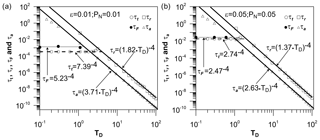Figure 8Model results displaying τt, τr, τF, and τa vs. TD on a log–log scale. τt, τr, τF, and τa denote the transition time, the reflection time, the fracture time, and the arrival time, respectively. The fit curves for reflection time and arrival time are represented by black lines, for transition time by dashed lines, and for fracture time by grey lines. (a) Numerical simulations with ε and PN=0.01; and (b) numerical simulations with ε and PN=0.05 (see Eqs. 20, 21, and 22).

As we showed earlier, it does not make sense to discuss the occurrence of bilinear flow after the pressure front has already arrived at the fracture tip. Nevertheless, the results show (Fig. 8) that the reflection time τr (related to the flow rate calculated in the well) is greater than the arrival time τa (related to the moment at which the isobars reach the fracture tip). It means that the reciprocal of dimensionless flow rate calculated in the well is proportional to the fourth root of time even when the pressure front has already reached the fracture tip.

4 Discussion

Dimensionless time was not defined using the conventional definition tD; instead, a modified definition τ presented by Ortiz R. et al. (2013) was used. It turned out to be convenient in terms of interpreting the results for bilinear flow since it was possible to graph the behavior of 1∕qwD vs. τ for all dimensionless fracture conductivities TD in the same graph (Fig. 2).

As for the comparison between the coefficient A=2.6 obtained by us (Eq. 12) and the coefficient A=2.772 documented by Guppy et al. (1981b), we can observe a discrepancy between these results of approximately 6 %. This discrepancy can be considered rather low.

Some type curves bend clockwise and some others bend counterclockwise from the bilinear fit curve (Fig. 2). Among the cases of dimensionless fracture conductivities TD studied, the type curves that bend clockwise are TD=0.1, 0.3 and 1.1, and those that bend counterclockwise are TD=3.1, 6.3, 9.4, 20, 31, 50, and 100. Similar results were obtained by Ortiz R. et al. (2013) for the case of injection at a constant flow rate. For the interval of time utilized in the simulation, the behavior of 1∕qwD versus τ for dimensionless fracture conductivities TD=0.1 and 0.3 is identical to the behavior of an infinitely long fracture (master curve, orange line in Fig. 2) since the separation of the mentioned type curves from the master curve should occur at a time greater than the simulation time utilized here.

Our results concerning the propagation of isobars along the fracture and the matrix (Eqs. 14, 15) are similar to the results previously presented by Ortiz R. et al. (2013) regarding the migration of isobars. The values of αb obtained by us for PN=0.01 and 0.05 are quantitatively different from the values documented by them in 4.6 % and 5.8 %, respectively.

At times, shortly before the isobars reach the fracture tip, they exhibit an acceleration along the fracture (see Figs. 4, 6). Subsequently, once the isobars arrive at the fracture tip, they no longer progress through the matrix over a certain period of time. Afterward, they experience another acceleration along the fracture, with which the migration of isobars seems to approach a propagation proportional to the square root of time (see Fig. 4). An identical behavior was observed by Ortiz R. et al. (2013), and they attributed it to the reflection of isobars at the fracture tip, which makes sense and could be confirmed in this study. The acceleration nearby the fracture tip can be observed more clearly when analyzing the velocity along the fracture (see Fig. 7b, d). During the intervals of time used, the migration of isobars along the fracture experiences a constant deceleration, except when they approach the fracture tip. This deceleration is qualitatively identical for PN=0.01 and PN=0.05 (see Fig. 7a, c). It is evident that for all fixed dimensionless positions in the fracture and considering the same dimensionless fracture conductivity TD, the velocity viD is higher for low values of normalized isobars PN (see Fig. 7b, d). One reason of this observation is that once the deceleration begins, PN=0.01 propagates faster than PN=0.05 since the initial velocity (when the isobar leaves the well) of the isobar PN=0.01 is higher than the initial velocity of the isobar PN=0.05. This behavior is explained based on the fact that the pressure gradient between the well and the fracture is bigger when PN=0.01 is leaving the well than when PN=0.05 is leaving it. Furthermore, for all fixed dimensionless positions in the fracture and considering the same isobar PN, the velocity viD is higher for high dimensionless fracture conductivities (see Fig. 7b, d).

Using Eqs. (18) and (19), the migration of isobars along the fracture can be described as

$\begin{array}{}\text{(23)}& {x}_{\mathrm{iD}}={\left(\frac{{\mathit{\beta }}_{\mathrm{b}}}{{\mathit{\gamma }}_{\mathrm{b}}}\right)}^{-\mathrm{1}/\mathrm{3}}{\mathit{\tau }}^{\mathrm{1}/\mathrm{4}}.\end{array}$

Note that Eq. (23) has the same form that Eq. (14); thus

$\begin{array}{}\text{(24)}& {\left(\frac{{\mathit{\beta }}_{\mathrm{b}}}{{\mathit{\gamma }}_{\mathrm{b}}}\right)}^{-\mathrm{1}/\mathrm{3}}={\mathit{\alpha }}_{\mathrm{b}}{T}_{\mathrm{D}}.\end{array}$

It is possible to verify the validity of Eq. (24) by introducing the required values.

For the case of injection at a constant flow rate the results obtained by Ortiz R. et al. (2013) for the arrival time, the reflection time, and the transition time are similar to ours (see Table 1). It is worth noting that when using the expression ε and PN=0.01, it means that we are studying the case of the isobar PN=0.01, and we consider that for values of ε greater than 0.01, the bilinear flow ends. Note further that when considering ε and PN=0.05, we are studying the isobar PN=0.05 and we are using a value of ε=0.05 to determine the termination of bilinear flow, for all pertinent criteria.

Table 1Comparison of coefficients of fit equations for the arrival time, the reflection time, and the transition time, which have the form ${\mathit{\tau }}_{\mathrm{a}}={\left({c}_{\mathrm{a}}\cdot {T}_{\mathrm{D}}\right)}^{-\mathrm{4}}$, ${\mathit{\tau }}_{\mathrm{r}}={\left({c}_{\mathrm{r}}\cdot {T}_{\mathrm{D}}\right)}^{-\mathrm{4}}$, and ${\mathit{\tau }}_{\mathrm{t}}={\left({c}_{\mathrm{t}}\right)}^{-\mathrm{4}}$, respectively (see Fig. 8).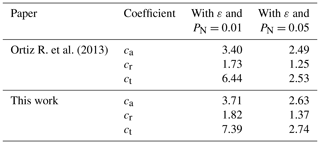When it comes to the criteria that consider the transition of 1∕qwD some observations can be made: (a) in the case of Fig. 8a the transition criterion is fulfilled up to a value of TD approximately of 2 and for values of TD above 3 the reflection criterion is fulfilled; and (b) in the case of Fig. 8b the transition criterion is fulfilled up to a value of TD of approximately 1.1 and for values of TD above 2 the reflection criterion is fulfilled. Note that for the case ε and PN=0.01 and $\mathrm{2}<{T}_{\mathrm{D}}<\mathrm{3}$ (see Fig. 8a), it is observed that values (non-filled circles) depart from the fit curve linked to the transition criterion and start converging toward the fit curve associated with the reflection criterion. A similar behavior is also observed for the case ε and PN=0.05 and $\mathrm{1.1}<{T}_{\mathrm{D}}<\mathrm{2}$ (see Fig. 8b). A comprehensive study is required to unravel more precisely what occurs within those ranges of TD. Based on their work, Ortiz R. et al. (2013) came to the same conclusion.

Ultimately, there are two ways to determine the termination of bilinear flow: (I) by numerically measuring the transient flow rate in the well and obtaining the transition time τt for low TD and the reflection time τr for high TD and (II) according to the migration of isobars along the fracture (not measurable in the well), obtaining the fracture time τF for low TD and the arrival time τa for high TD (see Table 2).

Table 2Criteria utilized to calculate the termination of bilinear flow. See discussion for the definition of the cases I and II.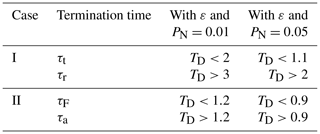In a follow-up study, it would be interesting to include the effect of fracture storativity and, utilizing an analogue method to that discussed in this work, investigate the behavior of a fracture with conductivity high enough to lead to fracture and formation linear flow.

## Application to well testing problems

In practical terms, when analyzing the transient flow rate in a well, only the transition time τt and the reflection time τr can be determined. With the current field methods, it is not possible to determine the termination of bilinear flow utilizing the progress of the pressure front along the fracture, although this is more physically reasonable. Nevertheless, the fracture length can be constrained indirectly, for instance by computing the time at which the pressure arrives at the fracture tip and its relation with respect to the reflection time. The relation between the arrival time τa and the reflection time τr is given by

$\begin{array}{}\text{(25)}& {\mathit{\tau }}_{\mathrm{a}}\cong \mathrm{0.0579}{\mathit{\tau }}_{\mathrm{r}}\end{array}$

for ε and PN=0.01 (see Fig. 8a), and

$\begin{array}{}\text{(26)}& {\mathit{\tau }}_{\mathrm{a}}\cong \mathrm{0.0736}{\mathit{\tau }}_{\mathrm{r}}\end{array}$

for ε and PN=0.05 (see Fig. 8b).

In the following, we present two artificial cases in which synthetic curves were constructed to illustrate how the measurements of the flow rate in wells during hydraulic tests at constant pressure are used to estimate or restrict the length of fractures with finite hydraulic conductivity (bilinear flow). The synthetic curves are not obtained from measurements of realistic well tests but computed utilizing the validated model concerning fractured porous geologic media, included in COMSOL Multiphysics® and in previous papers.

### Scenario A: high dimensionless fracture conductivities TD

We proceed to elaborate a method to estimate the fracture length using measurements of the flow rate in the well. This is motivated by its usefulness for cases with high TD in which the reflection criterion is applicable, i.e., provided that the isobar that is under study reaches the fracture tip while bilinear flow is still in progress. In this example, the values of the dimensional fracture conductivity TF as well as the fracture length 2xF are restricted by a synthetic curve representing the transient flow rate in the well. The synthetic curve is performed assuming that pw=1 MPa, pi=100 kPa, km=1µD, ${T}_{\mathrm{F}}=\mathrm{1.5}×{\mathrm{10}}^{-\mathrm{16}}$ m3, ${s}_{\mathrm{m}}={\mathrm{10}}^{-\mathrm{11}}$ Pa−1, ${\mathit{\eta }}_{\mathrm{F}}=\mathrm{2.5}×{\mathrm{10}}^{-\mathrm{4}}$ Pa s, and xF=23.81 m (see Fig. 9). The procedure is described in series of steps as follows:

1. Dimensionally graphing the reciprocal of flow rate vs. time. It is worthwhile noting that the counterclockwise separation of the synthetic curve (red line) from the bilinear fit curve (grey line) represents the moment of arrival of the pressure front at the fracture tip, defining the end of bilinear flow.

2. Calculate TF as is typically done (see, e.g., Guppy et al., 1981b), i.e., based on the slope of bilinear fit curve $\mathrm{1}/{q}_{\mathrm{w}}=m{t}^{\mathrm{1}/\mathrm{4}}$ (Eq. 12). The dimensional fracture conductivity is determined as follows:

$\begin{array}{}\text{(27)}& {T}_{\mathrm{F}}={\left(\frac{\mathrm{2.61}{\mathit{\eta }}_{\mathrm{F}}^{\mathrm{3}/\mathrm{4}}}{{k}_{\mathrm{m}}^{\mathrm{1}/\mathrm{4}}{s}_{\mathrm{m}}^{\mathrm{1}/\mathrm{4}}h\left({p}_{\mathrm{w}}-{p}_{\mathrm{i}}\right)m}\phantom{\rule{0.125em}{0ex}}\right)}^{\mathrm{2}}.\end{array}$

According to this example, TF is obtained as $\mathrm{1.5}×{\mathrm{10}}^{-\mathrm{16}}$ m3. This value is the same as the one employed to perform the synthetic curve.

3. Read from the graph the termination of bilinear flow defined by the separation of the curve that represents the 1∕qw measured in the well (red curve) from the curve proportional to t1∕4 (grey curve). This time corresponds to the reflection time. In practical terms, it is considered a calculation error in the separation of 5 %, which corresponds approximately to the visual estimation of the point at which these curves start departing from each other. In this case study, the reflection time tr is approximately 104 s.

4. Introduce the value of reflection time tr calculated in the previous step in the relation τa≅0.0736τr and obtain the arrival time of the isobars at the fracture tip. For the example at hand, ta=736 s.

5. Determine the value of Db from its definition:

$\begin{array}{}\text{(28)}& {D}_{\mathrm{b}}=\frac{{T}_{\mathrm{F}}^{\mathrm{2}}}{{k}_{\mathrm{m}}{\mathit{\eta }}_{\mathrm{F}}{s}_{\mathrm{m}}}.\end{array}$

For the present case study, taking into account the example and the parameters of the simulation, Db is obtained as 9 m4 s−1.

Introduce the value of ta, obtained at step 4, and the value of Db, calculated at step 5, in the equation of migration of isobars along the fracture (Eq. 16), and, in this way, calculate the fracture half-length. In this case, the isobar under study is PN=0.05; as a result the constant αb=2.23. Utilizing Eq. (16), we have

$\begin{array}{}\text{(29)}& {x}_{\mathrm{F}}={\mathit{\alpha }}_{\mathrm{b}}{\left({D}_{\mathrm{b}}{t}_{\mathrm{a}}\right)}^{\mathrm{1}/\mathrm{4}}.\end{array}$

When introducing the corresponding values of the considered example, xF is obtained as 20.12 m.

6. Finally, the fracture length is approximately 40.24 m (2xF). It can be noted that this result is slightly lower than 47.62 m, which is the value that denotes the real magnitude used to represent the synthetic curve. It is possible to obtain more accurate results by quantitatively calculating the separation between the curves of the considered example instead of visually estimating it. For instance, when calculating explicitly a counterclockwise 5 % separation of the synthetic curve (red line) from the bilinear fit curve (grey line), an arrival time of 865.5 s and a fracture length of 41.9 m are obtained.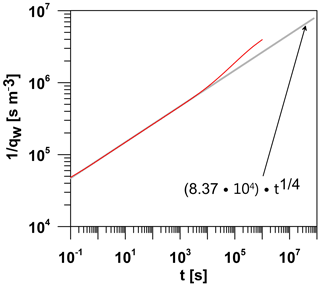Figure 91∕qw (s m−3) vs. t (s) on a log–log scale. The synthetic curve is represented by the red line and the bilinear fit curve is displayed with the grey line.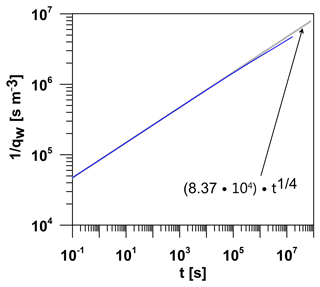Figure 101∕qw (s m−3) vs. t (s) on a log–log scale. The synthetic curve is represented by the blue line and the bilinear fit curve is indicated with the grey line.

### Scenario B: low dimensionless fracture conductivities TD

In the case of low TD, it is not possible to estimate the fracture length utilizing the bilinear flow theory, since this flow regime ends before the isobar under study arrives at the fracture tip. This is expressed in terms of the pressure field by the observation of the premature occurrence of a significant pressure change in the fracture tip. However, it is possible to restrict the minimum fracture length. In the following example, the values of the dimensional fracture conductivity TF as well as the minimum fracture length 2xF are constrained by a synthetic curve representing the transient flow rate in the well. This latter curve is computed assuming that pw=1 MPa, pi=100 kPa, km=1µD, ${T}_{\mathrm{F}}=\mathrm{1.5}×{\mathrm{10}}^{-\mathrm{16}}$ m3, ${s}_{\mathrm{m}}={\mathrm{10}}^{-\mathrm{11}}$ Pa−1, ${\mathit{\eta }}_{\mathrm{F}}=\mathrm{2.5}×{\mathrm{10}}^{-\mathrm{4}}$ Pa s, and xF=136.36 m (see Fig. 10). The procedure is outlined in the following steps:

1. Dimensionally graphing the reciprocal of flow rate vs. time.

2. Calculate the value of TF as commonly conducted in the related literature (see, e.g., Guppy et al., 1981b), i.e., based on the slope of bilinear fit curve $\mathrm{1}/{q}_{\mathrm{w}}=m{t}^{\mathrm{1}/\mathrm{4}}$ (Eq. 12). The dimensional fracture conductivity is determined as follows:

$\begin{array}{}\text{(30)}& {T}_{\mathrm{F}}={\left(\frac{\mathrm{2.61}{\mathit{\eta }}_{\mathrm{F}}^{\mathrm{3}/\mathrm{4}}}{{k}_{\mathrm{m}}^{\mathrm{1}/\mathrm{4}}{s}_{\mathrm{m}}^{\mathrm{1}/\mathrm{4}}h\left({p}_{\mathrm{w}}-{p}_{\mathrm{i}}\right)m}\phantom{\rule{0.125em}{0ex}}\right)}^{\mathrm{2}}.\end{array}$

According to this example, TF is obtained as $\mathrm{1.5}×{\mathrm{10}}^{-\mathrm{16}}$ m3. This value is the same as the one used to calculate the synthetic curve.

3. Read from the graph the termination of bilinear flow defined by the clockwise separation of the curve that represents the 1∕qw measured in the well (blue curve) from the curve proportional to t1∕4 (grey curve). This time is defined as transition time, and it is similar for all cases with low TD. Similar to the previous case, a calculation error in the separation of 5 % is considered, which corresponds approximately to the visual estimation of the point at which both curves start departing from each other. In this example, the transition time tt is approximately 106 s.

4. Introduce the value of transition time tt, calculated in the previous step, in the relation τa≅0.0736τt and obtain the fictitious arrival time of the isobars at the fracture tip. For the contemplated case example, ta=73 600 s.

5. Determine the value of Db from its definition:

$\begin{array}{}\text{(31)}& {D}_{\mathrm{b}}=\frac{{T}_{\mathrm{F}}^{\mathrm{2}}}{{k}_{\mathrm{m}}{\mathit{\eta }}_{\mathrm{F}}{s}_{\mathrm{m}}}.\end{array}$

In the context of the example at hand and considering the parameters of the simulation, Db is obtained as 9 m4 s−1.

Introduce the value of ta, obtained at step 4, and the value of Db computed at step 5, in the equation of migration of isobars along the fracture (Eq. 16) and, in this way, calculate the fictitious fracture half-length. In this case, the isobar under study is PN=0.05; as a consequence the constant αb=2.23. Utilizing Eq. (16) we have

$\begin{array}{}\text{(32)}& {x}_{\mathrm{F}}={\mathit{\alpha }}_{\mathrm{b}}{\left({D}_{\mathrm{b}}{t}_{\mathrm{a}}\right)}^{\mathrm{1}/\mathrm{4}}.\end{array}$

When incorporating the corresponding values of this example, xF is obtained as 63.62 m.

6. Finally, the minimum fracture length is approximately 127.23 m (2xF), whereas the real value used to represent the synthetic curve is 272.72 m.

In the cases described previously, the practical use of Eq. (16) to constrain the length of a fracture with finite conductivity has been demonstrated by analyzing the transient behavior of flow rate in the well during a hydraulic test at constant pressure.

The expressions obtained in this work for the end time of bilinear flow, the pressure propagation, and the bilinear diffusivity Db complement the limited theory that exists about data analysis from wells producing or injecting at constant pressure. The clarity and simplicity of these equations allow these to be used quickly to estimate the length of fractures with finite conductivity. The bilinear diffusivity Db, first introduced by Ortiz R. et al. (2013) for constant well flow rate and demonstrated in this work to also hold for the case of constant well pressure, could in principle be estimated in the laboratory by means of Eq. (16). In addition, this bilinear diffusivity allows, on the one hand, for a relatively uncomplicated comparison between finite-conductivity fractures. On the other hand, these equations could in one way or another be integrated into more general methods such as the transient rate analysis for the interpretation of production data. Finally, the diffusivity equations of pressure in the matrix and the fracture (Eqs. 16, 17) are also useful to reduce the associated risks related to induced seismicity generated by changes of pressure in fractured reservoirs or faults, as a consequence of massive fluid injection (e.g. Shapiro, 2015; Shapiro and Dinske, 2009). By knowing and understanding the physics behind the migration of isobars it is possible to minimize the associated risks with changes in pores pressure.

5 Conclusions

Numerical results obtained in this work corroborated the relation of proportionality previously presented by Guppy et al. (1981b) between the reciprocal of dimensionless flow rate 1∕qwD and the fourth root of dimensionless time τ during the bilinear flow regime for the case of injection at constant pressure in the well. Guppy et al. (1981b) obtained the proportionality factor A = 2.722 (Eq. 10), which is slightly greater than the factor obtained here A = 2.60 (Eq. 12). This discrepancy may be attributed to our finer spatial and temporal discretization in comparison with the discretization used by Guppy et al. (1981b).

The most significant findings of this work are as follows:

• i.

During the bilinear flow regime, the migration of isobars along the fracture is described as ${x}_{\mathrm{i}}\left(t\right)={\mathit{\alpha }}_{\mathrm{b}}\left({D}_{\mathrm{b}}t{\right)}^{\mathrm{1}/\mathrm{4}}$, where ${D}_{\mathrm{b}}={T}_{\mathrm{F}}^{\mathrm{2}}/{k}_{\mathrm{m}}{\mathit{\eta }}_{\mathrm{f}}{s}_{\mathrm{m}}$ (m4 s−1) is the effective hydraulic diffusivity of fracture during the bilinear flow regime. In addition, the migration of isobars in the matrix is given by ${y}_{\mathrm{i}}\left(t\right)={\mathit{\alpha }}_{\mathrm{m}}\left({D}_{\mathrm{m}}t{\right)}^{\mathrm{1}/\mathrm{2}}$, where ${D}_{\mathrm{m}}={k}_{\mathrm{m}}/\left({\mathit{\eta }}_{\mathrm{f}}{s}_{\mathrm{m}}$) (m2 s−1) denotes the hydraulic diffusivity of matrix. This simulation results are in line with the study conducted by Ortiz R. et al. (2013) for the case of wells injecting or producing at a constant flow rate.

• ii.

The termination of bilinear flow obtained from transient flow rate analysis is given by (a) the transition time τt (circumferences in Fig. 8 and Eq. 20), valid for low TD, and (b) the reflection time τr (squares in Fig. 8 and Eq. 21), valid for high TD.

• iii.

From the physical point of view, it is of interest to study the propagation of isobars along the fracture, for which the termination of bilinear flow has been found in this work to be given by (a) the fracture time τF (filled circles in Fig. 8 and Eq. 22), valid for low TD, and (b) the arrival time τa (triangles in Fig. 8), valid for high TD. However, this methodology may encounter technological obstacles in real field situations.

• iv.

A new methodology is presented to constrain the fracture length (Sect. 4), based on the end time of the bilinear flow and using Eq. (16), which describes the spatiotemporal evolution of the isobars along the fracture during the bilinear flow regime.

• v.

In terms of dimensionless parameters, the time at which a specific isobar arrives at the fracture tip is dependent only on TD (see Sect. 3.2.3 and τa in Fig. 8).

In agreement with Ortiz R. et al. (2013), we observe that the isobars exhibit a peak of acceleration shortly before they arrive at the fracture tip (Figs. 4, 6). This acceleration was verified by studying the velocity of isobars using the graphs viD vs. τ and viD vs. xiD (Fig. 7). We conclude that for a fixed dimensionless position in the fracture xiD, the velocity viD is higher for lower values of normalized isobars pN as well as for higher dimensionless fracture conductivities TD (see Fig. 7b, d).

Appendix A: Nomenclature
 A constant (Eq. 12) bF aperture of fracture (m) ca coefficient of fit equation for the arrival time (Table 1 and Fig. 8) cr coefficient of fit equation for the reflection time (Table 1 and Fig. 8) ct coefficient of fit equation for the transition time (Table 1 and Fig. 8) Db effective hydraulic diffusivity of fracture during bilinear flow regime (Eq. 16; m4 s−1) Dm hydraulic diffusivity of matrix (Eq. 17; m2 s−1) f(tD) transient behavior of pressure in the well (Eq. 11; Pa) h height of the open well section; fracture height (Eq. 5; m) kF fracture permeability (m2) km matrix permeability (m2) pi initial pressure of matrix and fracture (Eq. 5; Pa) PN normalized pressure difference (Eq. 13) pw constant injection pressure (Eq. 5; Pa) $p\left(x,y,t$) pressure at the position (x,y) in the fracture or the matrix at time t (Eq. 13; Pa) qw flow rate in the well (Eq. 5; m3 s−1) qwD dimensionless flow rate in the well (Eq. 5) qwDt dimensionless flow rate of type curves (Eqs. 20 and 21; Fig. 2) qwD∞ dimensionless flow rate of the master curve (Eq. 21; Fig. 2) qF(x,t) fluid flow between matrix and fracture (m2 s−1) sF specific storage capacity of fracture (Pa−1) sm specific storage capacity of matrix (Pa−1) t dimensional time (Eq. 7; s) ta dimensional arrival time (s) tD conventional dimensionless time (Eq. 7) TD dimensionless fracture conductivity (Eq. 6) TF fracture conductivity (Eq. 6; m3) viD dimensionless velocity of isobars along the fracture (Eqs. 18 and 19) xy spatial coordinates along and normal to the fracture with origin at the well (Eqs. 8 and 9, respectively; m) xF fracture half-length (Eq. 6; m) xDyD dimensionless coordinates (Eqs. 8 and 9, respectively) xiDyiD dimensionless distances of isobars from the well (along the xD and yD axis (Eqs. 14 and 15, respectively) xiDf dimensionless propagation of migration fit curves (Eq. 22; Fig. 4) xiDt dimensionless propagation of migration type curves (Eq. 22; Fig. 4) αb constant for pressure diffusion in the fracture during bilinear flow (Eqs. 14 and 16) αm constant for pressure diffusion in the matrix (Eqs. 15 and 17) βb constant for velocity in the fracture depending on time (Eq. 18) γb constant for velocity in the fracture depending on space (Eq. 19) δ constant (Eq. 11) ε quantification of error in the termination of bilinear flow (Eqs. 20, 21, and 22; Fig. 8) ηf dynamic fluid viscosity (Pa s) τ dimensionless time (Eq. 7) τa dimensionless arrival time (Fig. 8) τF dimensionless fracture time (Fig. 8) τr dimensionless reflection time (Fig. 8) τt dimensionless transition time (Fig. 8)
Data availability
Data availability.

The underlying research data are the result of computational experiments that are described in detail throughout the paper and the data are therefore reproducible by readers. Our work does not include experimental data from laboratory or field measurements. Where necessary, the computational model setup, from which the research data can be obtained, can be provided by the authors upon request.

Author contributions
Author contributions.

PIPD was responsible for the numerical model and simulations, the analysis of results, the preparation of the draft, and the final paper. AEOR contributed to the theoretical underpinning, presentation of results, and preparation of the draft and final paper. EMR contributed towards the theoretical underpinning in composing the numerical model, analysis and presentation of results, and preparation of the draft and final paper.

Competing interests
Competing interests.

The authors declare that they have no conflict of interest.

Special issue statement
Special issue statement.

Acknowledgements
Acknowledgements.

Accomplishing this assignment was only possible through the international collaboration between the Department of Chemical and Environmental Engineering – Universidad Técnica Federico Santa María (Chile) and the Department of Geothermics and Information Systems – Leibniz Institute for Applied Geophysics (Germany). This scientific cooperation has been extended to the Federal Institute for Geosciences and Natural Resources (BGR, Germany) during the completion phase of this work. We are grateful to the BGR for facilitating a cooperative framework. We would particularly like to thank the two anonymous reviewers and editor for a critical reading and detailed revision of the paper. Their useful suggestions and thorough corrections significantly helped to improve this work.

Review statement
Review statement.

This paper was edited by Peter Eichhubl and reviewed by two anonymous referees.

References

Arps, J. J.: Analysis of Decline Curves, Trans. AIME, 160, 228–247, https://doi.org/10.2118/945228-G, 1945.

Agarwal, R. G., Carter, R. D., and Pollock, C. B.: Evaluation and Performance Prediction of Low-Permeability Gas Wells Stimulated by Massive Hydraulic Fracturing, J. Pet. Technol., 31, 362–372, https://doi.org/10.2118/6838-PA, 1979.

Barenblatt, G., Zheltov, I., and Kochina, I.: Basic concepts in the theory of seepage of homogeneous liquids in fissured rocks [strata], J. Appl. Math. Mech., 24, 1286–1303, https://doi.org/10.1016/0021-8928(60)90107-6, 1960.

Berumen, S., Samaniego, F., and Cinco, H.: An investigation of constant-pressure gas well testing influenced by high-velocity flow, J. Petrol. Sci. Eng., 18, 215–231, https://doi.org/10.1016/S0920-4105(97)00014-4, 1997.

Cinco-Ley, H. and Samaniego-V., F.: Transient Pressure Analysis for Fractured Wells, J. Pet. Technol., 33, 1749–1766, https://doi.org/10.2118/7490-PA, 1981.

Cinco L., H., Samaniego V., F., and Dominguez A., N.: Transient Pressure Behavior for a Well With a Finite-Conductivity Vertical Fracture, Soc. Petrol. Eng. J., 18, 253–264, https://doi.org/10.2118/6014-PA, 1978.

Da Prat, G.: Well test analysis for fractured reservoir evaluation, no. 27 in Developments in Petroleum Science, Elsevier Science Publishers B.V., Amsterdam, 1990.

Dejam, M., Hassanzadeh, H., and Chen, Z.: Semi-analytical solution for pressure transient analysis of a hydraulically fractured vertical well in a bounded dual-porosity reservoir, J. Hydrol., 565, 289–301, https://doi.org/10.1016/j.jhydrol.2018.08.020, 2018.

Earlougher Jr., R. C.: Advances in Well Test Analysis, Henry L. Doherty series; Monograph (Society of Petroleum Engineers of AIME), v. 5., Henry L. Doherty Memorial Fund of AIME, New York, 1977.

Ehlig-Economides, C. A.: Well test analysis for wells produced at a constant pressure, Dissertation, Stanford University, 1979.

Fetkovich, M. J.: Decline Curve Analysis Using Type Curves, J. Pet. Technol., 32, 1065–1077, https://doi.org/10.2118/4629-PA, 1980.

Gidley, J., Holditch, S. A., Nierode, D. E., Ralph, W., and Veatch, J.: Recent Advances in Hydraluic Fracturing, Henry L. Doherty series; Monograph (Society of Petroleum Engineers of AIME), Vol. 12, 1990.

Guppy, K. H., Cinco-Ley, H., and Ramey, H. J.: Effect of Non-Darcy Flow on the Constant-Pressure Production of Fractured Wells, Soc. Petrol. Eng. J., 21, 390–400, https://doi.org/10.2118/9344-PA, 1981a.

Guppy, K. H., Cinco-Ley, H., and Ramey, H. J.: Transient Flow Behavior of a Vertically Fractured Well Producing at Constant Pressure, Soc. Petrol. Eng. J., SPE 9963, 1981b.

Guppy, K. H., Kumar, S., and Kagawan, V. D.: Pressure-Transient Analysis for Fractured Wells Producing at Constant Pressure, SPE Formation Eval., 3, 169–178, https://doi.org/10.2118/13629-PA, 1988.

He, Y., Cheng, S., Rui, Z., Qin, J., Fu, L., Shi, J., Wang, Y., Li, D., Patil, S., Yu, H., and Lu, J.: An improved rate-transient analysis model of multi-fractured horizontal wells with non-uniform hydraulic fracture properties, Energies, 11, 1–17, https://doi.org/10.3390/en11020393, 2018.

Heidari Sureshjani, M. and Clarkson, C. R.: Transient linear flow analysis of constant-pressure wells with finite conductivity hydraulic fractures in tight/shale reservoirs, J. Petrol. Sci. Eng., 133, 455–466, https://doi.org/10.1016/j.petrol.2015.06.036, 2015.

Houzé, O., Viturat, D., and Fjaere, O. S.: Dynamic Data Analysis – v.5.20, Edited by KAPPA Engineering, available at: https://www.kappaeng.com/downloads/ddabook (last access: 21 July 2020), 2018.

Kutasov, I. M. and Eppelbaum, L. V.: Drawdown test for a stimulated well produced at a constant bottomhole pressure, First Break, 23, 25–28, 2005.

Locke, C. D. and Sawyer, W. K.: Constant Pressure Injection Test in a Fractured Reservoir-History Match Using Numerical Simulation and Type Curve Analysis, in: Fall Meeting of the Society of Petroleum Engineers of AIME, Dallas, Texas, 28 September–1 October 1975.

Nashawi, I. S.: Constant-pressure well test analysis of finite-conductivity hydraulically fractured gas wells influenced by non-Darcy flow effects, J. Petrol. Sci. Eng., 53, 225–238, https://doi.org/10.1016/j.petrol.2006.06.006, 2006.

Nashawi, I. S. and Malallah, A. H.: Well test analysis of finite-conductivity fractured wells producing at constant bottomhole pressure, J. Petrol. Sci. Eng., 57, 303–320, https://doi.org/10.1016/j.petrol.2006.10.009, 2007.

Ortiz R., A. E., Jung, R., and Renner, J.: Two-dimensional numerical investigations on the termination of bilinear flow in fractures, Solid Earth, 4, 331–345, https://doi.org/10.5194/se-4-331-2013, 2013.

Pratikno, H., Rushing, J. A., and Blasingame, T. A.: Decline Curve Analysis Using Type Curves – Fractured Wells, in: SPE Annual Technical Conference and Exhibition, Society of Petroleum Engineers, Denver, Colorado, 5–8 October, https://doi.org/10.2118/84287-MS, 2003.

Samaniego-V., F. and Cinco-Ley, H.: Transient Pressure Analysis for Variable Rate Testing of Gas Wells, in: Low Permeability Reservoirs Symposium, Denver, Colorado, 15–17 April, 1991.

Shapiro, S. A.: Fluid-Induced Seismicity, Cambridge University Press, Cambridge, England, 1–276, 2015.

Shapiro, S. A. and Dinske, C.: Fluid-induced seismicity: Pressure diffusion and hydraulic fracturing, Geophys. Prospect., 57, 301–310, https://doi.org/10.1111/j.1365-2478.2008.00770.x, 2009.

Silva-López, D., Solano-Barajas, R., Turcio, M., Vargas, R. O., Manero, O., Balankin, A. S., and Lira-Galeana, C.: A generalization to transient bilinear flows, J. Petrol. Sci. Eng., 167, 262–276, https://doi.org/10.1016/j.petrol.2018.03.109, 2018.

Torcuk, M. A., Kurtoglu, B., Fakcharoenphol, P., and Kazemi, H.: Theory and Application of Pressure and Rate Transient Analysis in Unconventional Reservoirs, in: SPE Annual Technical Conference and Exhibition, Society of Petroleum Engineers, New Orleans, Louisiana, 30 September–2 October, https://doi.org/10.2118/166147-MS, 2013.

Wang, M., Fan, Z., Xing, G., Zhao, W., Song, H., and Su, P.: Rate decline analysis for modeling volume fractured well production in naturally fractured reservoirs, Energies, 11, 1–21, https://doi.org/10.3390/en11010043, 2018.

Wattenbarger, R. A., El-Banbi, A. H., Villegas, M. E., and Maggard, J. B.: Production Analysis of Linear Flow Into Fractured Tight Gas Wells, in: SPE Rocky Mountain Regional/Low-Permeability Reservoirs Symposium, Society of Petroleum Engineers, Denver, Colorado, 5–8 April, https://doi.org/10.2118/39931-MS, 1998.

Weir, G. J.: Single-phase flow regimes in a discrete fracture model, Water Resour. Res., 35, 65–73, https://doi.org/10.1029/98WR02226, 1999.